## Mathematics Seminar

Department of Mathematics, University of Ostrava

Past talks (2022)
 1. February 15, 2022 Pasha Zusmanovich (University of Ostrava) $$\omega$$-associative algebras Abstract: $$\omega$$-Lie algebras are certain generalizations of (ordinary) Lie algebras, where the Jacobi identity is twisted by a bilinear form. They occurred in physics in an attempt to deform the Lie algebra structures in a meaningful, in a physical sense, way ([N]). Recently an associative counterpart of those algebras arose in an entirely different situation, in differential geometry ([BKM]). So a natural problem arises to build a structure theory of $$\omega$$-associative algebras. One can expect this can be done similarly with the $$\omega$$-Lie case ([Z]), using rudiments of the structure theory of associative algebras, and an elementary linear algebra. Literature: [N] P. Nurowski, Deforming a Lie algebra by means of a 2-form, J. Geom. Phys. 57 (2007), 1325-1329 [Z] P. Zusmanovich, $$\omega$$-Lie algebras, J. Geom. Phys. 60 (2010), no.6-8, 1028-1044 [BKM] A.V. Bolsinov, A.Yu. Konyaev, V.S. Matveev, Applications of Nijenhuis geometry III: Frobenius pencils and compatible non-homogeneous Poisson structures; arXiv:2112.09471 2. March 8, 2022 Pasha Zusmanovich (University of Ostrava) Iterative correlation matrices Abstract: The method of iterative correlation matrices is widely used in applied statistics, but its rigorous justification is lacking. I will present the mathematical and computational aspects of this problem. Literature: C.-H. Chen, Generalized association plots: information visualization via iterative generated correlation matrices, Statistica Sinica 12 (2002), 7-29 J.B. Kruskal, A theorem about CONCOR, Technical Report MH 2C-571, Bell Laboratories, 1978 3. March 22, 2022 Ladislav Mišík (University of Ostrava) Limit of sequence with respect to a measure on $$\mathbb N$$ Abstract: Limit of sequences is a basic concept in mathematical analysis. In this talk we present it in more details using another basic concept of analysis, measure on sets of positive integers. A key role in our considerations is played by the concept of a degree of convergence of a given sequence to a given point with respect to a particular measure on the set of positive integers, as a number in the interval $$[0,1]$$. We study its properties depending on properties of the chosen measure. It appears that standard limits and their known generalizations (convergence with respect to a filter or ideal) are extremal special cases in our approach. Slides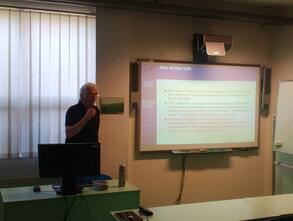4. April 5, 2022 Ondřej Turek (University of Ostrava) Representations of numbers as sums and products of continued fractions Abstract: The talk will be devoted to representations of real numbers as sums and products of continued fractions whose partial quotients obey certain restrictions.5. April 19, 2022 Živorad Tomovski (University of Ostrava) Fractional characteristic functions and fractional moments of random variables Abstract: In this talk we will consider a fractional variant of the characteristic function of a random variable. It exists on the real whole line, where it is uniformly continuous. We show that fractional moments can be expressed in terms of Riemann-Liouville integrals and derivatives of the fractional characteristic function. Fractional moments are of interest in particular for distributions whose integer moments do not exist. Some illustrative examples for classical and fractional pdf's will be presented. Literature: Ž. Tomovski, R. Metzler, S. Gerhold, Fractional characteristic functions, and a fractional calculus approach for moments of random variables, Frac. Calc. Appl. Anal., to appear Slides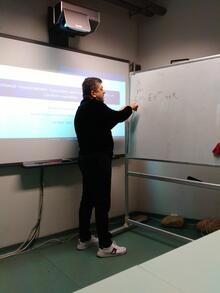6-7. April 26, 28, 2022 Paweł Gładki (University of Silesia, Katowice) Selected applications of multivalued algebras to the algebraic theory of quadratic forms Abstract: Hypergroups are objects like groups but with addition taking possibly many values. Likewise, hyperrings and hyperfields are objects similar to rings and fields, but with multivalued addition. Hyperfields provide a convenient tool in axiomatizing the algebraic theory of quadratic forms and in these talks we shall focus on three such applications. Firstly, we shall show how Witt equivalence of fields can be conveniently expressed in the language of hyperfields and will present some recent results on Witt equivalence of function fields over global and local fields. Secondly, we shall show how orderings of higher level can be defined for hyperrings and hyperfields, and, consequently, how they can be used to provide an axiomatic framework to study forms of higher order. Finally, we shall define the category of, so called, presentable fields and define their Witt rings, thus providing yet another machinery to study quadratic forms over fields. The results presented in these talks were obtained jointly with Murray Marshall and Krzysztof Worytkiewicz. Slides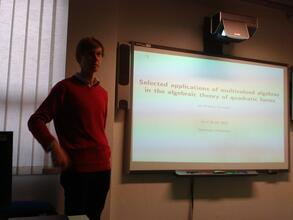8. May 3, 2022 Ly Hong Hai (University of Ostrava) The Neumann Laplacian in a perturbed domain Abstract: We consider a domain with a small compact set of zero Lebesgue measure removed. Our main result concerns the spectrum of the Neumann Laplacian defined on such domain. We prove that the spectrum of the Laplacian converges in the Hausdorff distance sense to the spectrum of the Laplacian defined on the unperturbed domain. Slides9. May 4, 2022 Alexander Šostak (University of Latvia) Fuzzy concept lattices in fuzzy rough set theory: basics of the theory and applications Abstract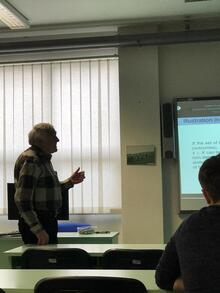10. May 10, 2022 Katarzyna Górska (Institute of Nuclear Physics, Kraków) Subordination and memory depend kinetics Abstract: Motivated by the paper authored by A.V. Chechkin and I.M. Sokolov entitled "On relation between generalized diffusion and subordination schemes" published in Phys. Rev. E 103, 032133 (2021), we study subordination using methods of classical mathematical analysis. Our main tool, namely the Efross theorem emerging from the Laplace-Borel convolution, directly leads to integral decomposition whose probabilistic interpretation (if allowed) guides us to subordination which encodes memory effects. We show that splitting the integral decompositions on the parent and leading processes is an ambiguous procedure. However, if the parent process is fixed then its partner leading process appears to be unique. Commonly assumed parent process given by the Brownian motion is by no means the only one possibility. We illustrate this on the example of the generalized Cattaneo equation for which we construct two distinct subordinations. For the first of them the parent process is given by the Gaussian while for the second one we get the Cattaneo-Vernotte, that is the finite speed, propagation. Non-uniqueness in the choice of the parent and leading processes is better visible in the case of standard non-Debye relaxation patterns. For example, the Havriliak-Negami relaxation can be expressed as the subordination either of the Debye model or the Cole-Davidson one. Slides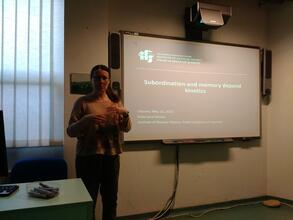11. May 17, 2022 Tobiasz Pietrzak (Institute of Nuclear Physics, Kraków) Ideal clock as the fundamental relativistic rotator Abstract: The clock hypothesis is the fundamental assumption in the special and general theory of relativity and says that duration, measured by an ideal clock, is proportionate to the length of its worldline. To test the hypothesis, a model of an ideal clock as a dynamical system described by position, a single null direction, and two parameters mass and length, with the additional condition that Casimir invariants of the Poincare group of this system are parameters with fixed numerical values rather than arbitrary constants of motion was introduced by Professor Staruszkiewicz. I will present the model and show why it is defective as a dynamical system. Slides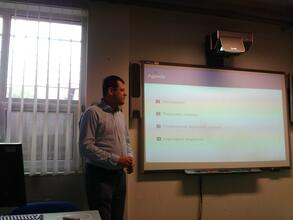12. June 13, 2022 Přemysl Jedlička (Czech University of Life Sciences, Prague) Central nilpotency of skew braces Abstract: Skew braces are algebraic structures related to the solutions of the set-theoretic quantum Yang-Baxter equation. We develop the central nilpotency theory for such algebraic structures in the sense of Freese-McKenzie and we compare the universal algebraic notion of central nilpotency with the traditional notion of right and left nilpotency. Slides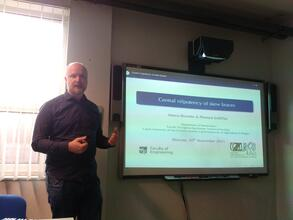13. September 19, 2022 Sayat Nurzhauov (Al-Farabi Kazakh National University) Tortkara and other nonassociative algebras Abstract: We compute dimensions of multilinear components of free algebras in some varieties of nonassociative algebras, including Tortkara and left weak Leibniz algebras. Some of the results are supported by computer computations in Haskell.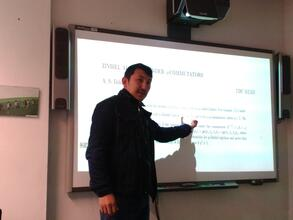14. October 3, 2022 Pasha Zusmanovich (University of Ostrava) Baer's theorem, infinite matrices, and all that Abstract: If two matrix algebras of the size $$n \times n$$ and $$m \times m$$ are isomorphic, then the matrices are of the same size. This trivial result which amounts to the implication $$n^2 = m^2 \Rightarrow n = m$$ is not so obvious if we are passing to matrices of infinite size. In fact, dealing with matrices of infinite size requires a great care (the product may be not defined, and if defined, may be not associative!), and a more appropriate language in this setting is those of endomorphisms of vector spaces. The classical Baer theorem from 1950's states that if endomorphism rings of infinite-dimensional vector spaces over a division ring are isomorphic, then the vector spaces themselves are isomorphic, and there are numerous generalizations and variations of this result in different directions. I will discuss yet another variation, which requires only elementary linear algebra. 15. November 10, 2022 Jan Novák (Czech Technical University, Prague) Plabic graphs in physics Abstract: One of the quickly developing mathematical disciplines is algebraic geometry. We study plabic (planar bicolored) graphs. LE-diagrams and decorated permutations will be defined in the first part of the talk. We mention that these three notions are interconnected. Further important terms will be the formal boundary measurements and the winding index. The amplituhedron $$\mathscr{A}_{n,k,m}$$ is the image in the Grassmannian $$Gr_{k(k+m)}$$ of the totally nonnegative part of $$Gr_{k,n}$$ under a totally positive linear map. It was introduced in 2013 in order to give a geometric basis for the computation in supersymmetric quantum field theory. We show a completely different possible usage of plabic graphs in applications to quantum gravity (ring paradigm), which is a work in progress. We will make a note at the end that algebraic geometry is frequently used in string theory as well. Slides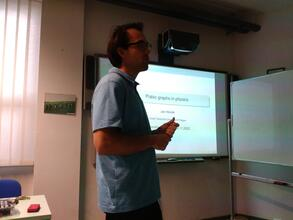16. December 1, 2022 Anatolij Prykarpatsky (Krakow Polytechnical University and Lviv Polytechnical University) On the classical A. Buhl problem, its G. Pfeiffer solution and its connection with completely integrable heavenly type dynamical systems Abstract   Slides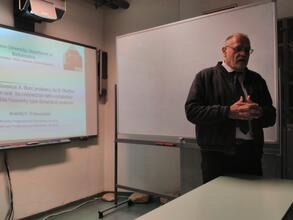17. December 8, 2022 Alexander Zuevsky (Institute of Mathematics, Czech Academy of Sciences, Prague) Vertex algebra bicomplexes, continual Lie algebras, and reduction cohomology Abstract: The orthogonality and double grading conditions on the bicomplex structure of a vertex algebra cohomology endow it with the structure of a bigraded differential algebra with respect to a natural multiplication. Its generators and commutation relations form a continual Lie algebra with the root space associated to a vertex algebra, and provide non-vanishing cohomological invariants. We will introduce the notion of the reduction cohomology of Riemann surfaces in terms of vertex operator algebra n-point correlation functions. Algebraic, geometrical, and cohomological meanings of reduction formulas will be clarified.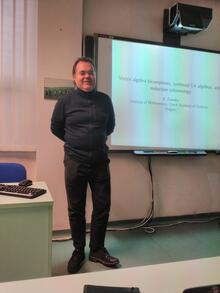18. December 15, 2022 (online) Petr Kučera (Czech Technical University, Prague) Mathematical models of flow and heat transfer with mixed boundary conditions Abstract: In the lecture, we deal with some models of flow and heat transfer in incompressible fluids, e.g. the so-called complete system of the Navier-Stokes equations or the Boussinesq equations. We present some results for these models where we prescribe the mixed boundary conditions as the solvability of these models and other qualitative properties.

Created: Sat Feb 26 2022